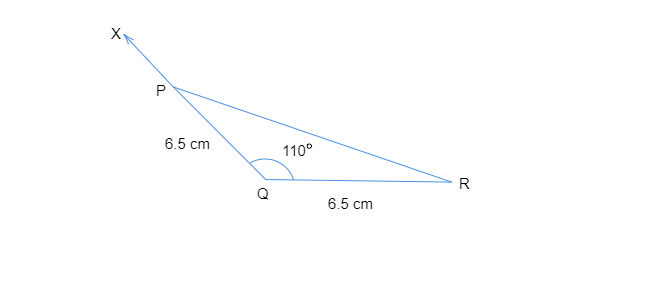# Construct an isosceles triangle in which the lengths of each of its equal sides is $6.5\ cm$ and the angle between them is $110^{\circ}$.

To do: To construct an isosceles triangle in which the lengths of each of its equal sides are $6.5\ cm$ and the angle between them is $110^{\circ}$.

Steps of construction:
• Let us draw a line segment $QR=6.5\ cm$.
• At the point $Q$, let us draw a ray $QX$ making an angle of $110^{\circ}$ with $QR$ such that $\angle XQR=110^{\circ}$.
• Assuming $Q$ as the center, let us draw an arc of radius $6.5\ cm$ that cuts $QX$ at point $P$.
• Now let us join $PR$ to get the required triangle.Thus, ​​​​​​$\triangle PQR$  is the required triangle.